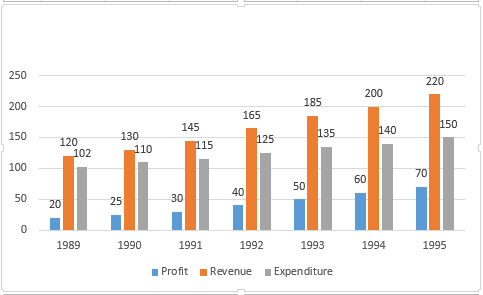### CAT 1996 Question Paper Question 46

Instructions

Answer the questions based on the following information.Question 46

# The average revenue collected in the given 7 years is approximately

Solution

Average will be = $$(120 + 130 + 145 + 165 + 185 + 200 + 220) \div 7 = 166$$ (nearly)

• All Quant CAT Formulas and shortcuts PDF
• 30+ CAT previous papers with solutions PDF